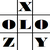anything in Excel...

It's Excel-o-lozy...

### Formula - Rounding to hundreds ('00) or thousands ('000)

U r thinking wats the pro thingy in "Round" formula....
Ok, m sure u wont think it after reading followings...

How do u round a number say "12345.67"?

Do u do round like...
=round(12345.67,0) where result is 12346, or
= roundup(12345.67,0) where result is 12346, or
= rounddown(12345.67,0) where result is 12345

ok, new thing, u can round like...
=round(12345.67,) where result is 12346, or
= roundup(12345.67,) where result is 12346, or
= rounddown(12345.67,) where result is 12345
i.e. without entering zero (0) after comma...

Nw u r thinking wat the hell... wat the pro thingy in it...

ok, tell me hw u round in hundreds ('00) or thousands ('000), if u wish to?????
Ok, dont think...
see, for rounding to, say 2 digits after decimal, v place 2 after comma,
same way to round, say 2 digits before decimal, v ll place -2 after comma...like...
=round(12345.67,-2) where result is 12300, or
= roundup(12345.67,-2) where result is 12400, or
= rounddown(12345.67,-2) where result is 12300...